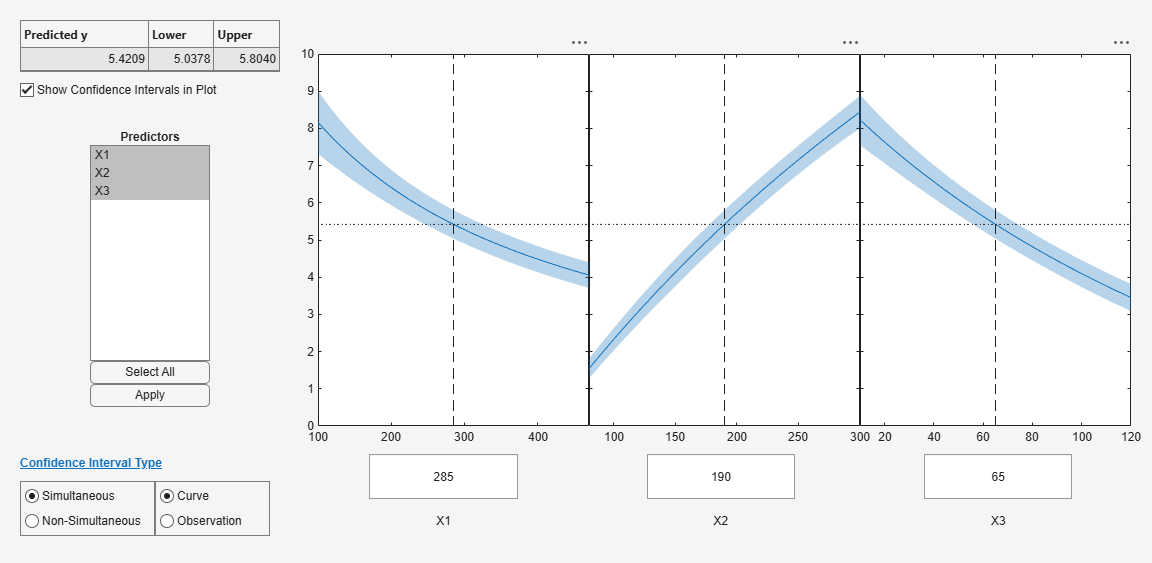Documentation

### This is machine translation

Mouseover text to see original. Click the button below to return to the English version of the page.

Note: This page has been translated by MathWorks. Click here to see
To view all translated materials including this page, select Country from the country navigator on the bottom of this page.

## Nonlinear Regression Workflow

This example shows how to do a typical nonlinear regression workflow: import data, fit a nonlinear regression, test its quality, modify it to improve the quality, and make predictions based on the model.

### Step 1. Prepare the data.

Load the `reaction` data.

`load reaction`

Examine the data in the workspace. `reactants` is a matrix with 13 rows and 3 columns. Each row corresponds to one observation, and each column corresponds to one variable. The variable names are in `xn`:

`xn`
```xn = 3x10 char array 'Hydrogen ' 'n-Pentane ' 'Isopentane' ```

Similarly, `rate` is a vector of 13 responses, with the variable name in `yn`:

`yn`
```yn = 'Reaction Rate' ```

The `hougen.m` file contains a nonlinear model of reaction rate as a function of the three predictor variables. For a 5-D vector $b$ and 3-D vector $x$,

`$hougen\left(b,x\right)=\frac{b\left(1\right)x\left(2\right)-x\left(3\right)/b\left(5\right)}{1+b\left(2\right)x\left(1\right)+b\left(3\right)x\left(2\right)+b\left(4\right)x\left(3\right)}$`

As a start point for the solution, take `b` as a vector of ones.

`beta0 = ones(5,1);`

### Step 2. Fit a nonlinear model to the data.

```mdl = fitnlm(reactants,... rate,@hougen,beta0)```
```mdl = Nonlinear regression model: y ~ hougen(b,X) Estimated Coefficients: Estimate SE tStat pValue ________ ________ ______ _______ b1 1.2526 0.86702 1.4447 0.18654 b2 0.062776 0.043562 1.4411 0.18753 b3 0.040048 0.030885 1.2967 0.23089 b4 0.11242 0.075158 1.4957 0.17309 b5 1.1914 0.83671 1.4239 0.1923 Number of observations: 13, Error degrees of freedom: 8 Root Mean Squared Error: 0.193 R-Squared: 0.999, Adjusted R-Squared 0.998 F-statistic vs. zero model: 3.91e+03, p-value = 2.54e-13 ```

### Step 3. Examine the quality of the model.

The root mean squared error is fairly low compared to the range of observed values.

`[mdl.RMSE min(rate) max(rate)]`
```ans = 1×3 0.1933 0.0200 14.3900 ```

Examine a residuals plot.

`plotResiduals(mdl)`The model seems adequate for the data.

Examine a diagnostic plot to look for outliers.

`plotDiagnostics(mdl,'cookd')`Observation `6` seems out of line.

### Step 4. Remove the outlier.

Remove the outlier from the fit using the `Exclude` name-value pair.

```mdl1 = fitnlm(reactants,... rate,@hougen,ones(5,1),'Exclude',6)```
```mdl1 = Nonlinear regression model: y ~ hougen(b,X) Estimated Coefficients: Estimate SE tStat pValue ________ ________ ______ _______ b1 0.619 0.4552 1.3598 0.21605 b2 0.030377 0.023061 1.3172 0.22924 b3 0.018927 0.01574 1.2024 0.26828 b4 0.053411 0.041084 1.3 0.23476 b5 2.4125 1.7903 1.3475 0.2198 Number of observations: 12, Error degrees of freedom: 7 Root Mean Squared Error: 0.198 R-Squared: 0.999, Adjusted R-Squared 0.998 F-statistic vs. zero model: 2.67e+03, p-value = 2.54e-11 ```

The model coefficients changed quite a bit from those in `mdl`.

### Step 5. Examine slice plots of both models.

To see the effect of each predictor on the response, make a slice plot using `plotSlice(mdl)`.

`plotSlice(mdl) ``plotSlice(mdl1) `The plots look very similar, with slightly wider confidence bounds for `mdl1`. This difference is understandable, since there is one less data point in the fit, representing over 7% fewer observations.

### Step 6. Predict for new data.

Create some new data and predict the response from both models.

```Xnew = [200,200,200;100,200,100;500,50,5]; [ypred yci] = predict(mdl,Xnew)```
```ypred = 3×1 1.8762 6.2793 1.6718 ```
```yci = 3×2 1.6283 2.1242 5.9789 6.5797 1.5589 1.7846 ```
`[ypred1 yci1] = predict(mdl1,Xnew)`
```ypred1 = 3×1 1.8984 6.2555 1.6594 ```
```yci1 = 3×2 1.6260 2.1708 5.9323 6.5787 1.5345 1.7843 ```

Even though the model coefficients are dissimilar, the predictions are nearly identical.

Download ebook